# Penetrable square well model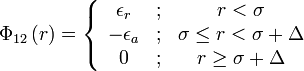$\Phi_{12}\left( r \right) = \left\{ \begin{array}{ccc} \epsilon_r & ; & r < \sigma \\ - \epsilon_a & ; &\sigma \le r < \sigma + \Delta\\ 0 & ; & r \ge \sigma + \Delta\end{array} \right.$
where$\Phi_{12}(r)$ is the intermolecular pair potential,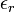$\epsilon_r$ is the hight of the repulsive core and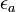$\epsilon_a$ is the attractive well depth,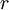$r$ is the distance between site 1 and site 2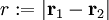$r := |\mathbf{r}_1 - \mathbf{r}_2|$.